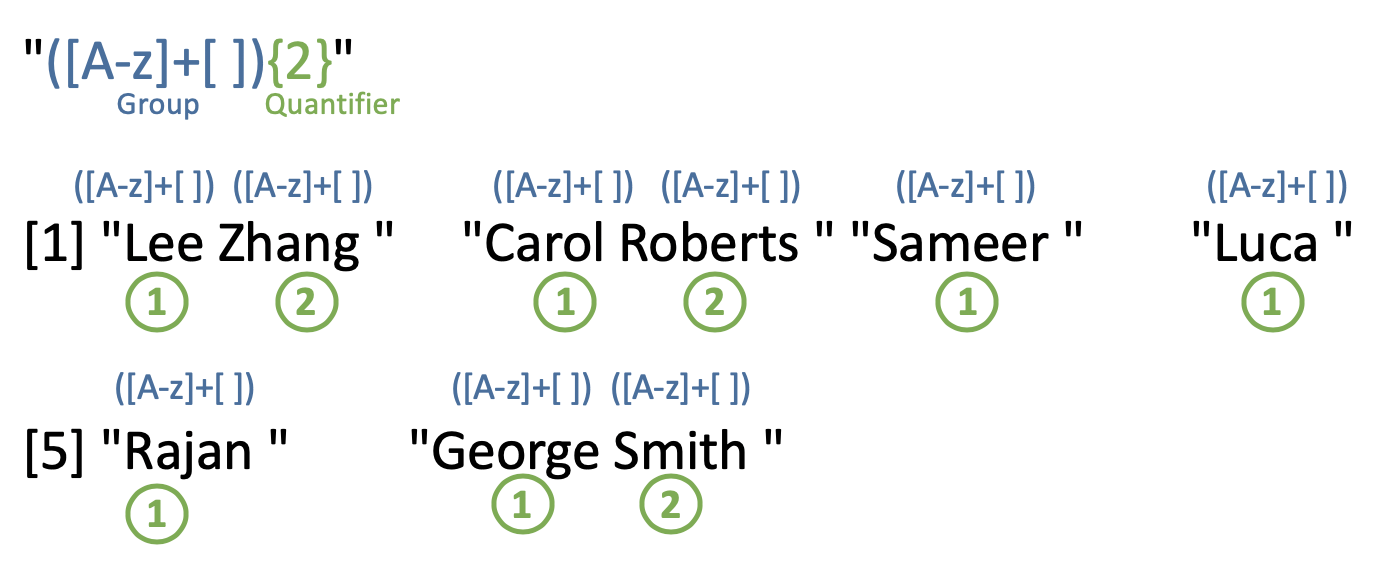# 14 Quantifiers

#### by Chitra Venkatesh

Quantifiers quantify the number of instances of a character, group or character class, denoted as P in the table below. Your quantifier should be placed after the character/group/character class that is being quantified. We will also explain what groups are in this context.

Quantifier Description
P* 0 or more instances of P
P+ 1 or more instances of P
P? 0 or 1 instance of P
P{m} Exactly m instances of P
P{m,} At least m instances of P
P{m,n} Between m and n instances of P

In the following example, let us try to extract all those names that contain more than 4 characters and less than 7 characters.

student_names <- c("Lee", "Carol", "Sameer", "Luca", "Rajan", "George Jr.")

str_extract(student_names, regex("^[A-z]{5,7}$")) #>  NA "Carol" "Sameer" NA "Rajan" NA In the above example, we used anchors ^ and $ to indicate an exact match. In absence of which a substring of George Jr. also gets displayed.

Let’s try to detect names of those individuals with one or more e or u.

str_detect(student_names, regex("[eu]+"))
#>   TRUE FALSE  TRUE  TRUE FALSE  TRUE

In the last example, if we want to extract names that contain e or u we could follow this simple implementation . Points to note here:

• Character set [eu] could appear 1 or more times so we use quantifier +.

• .* matches 0 or any number of characters where . is a wildcard dot and * represents the quantifier 0 or many

• Pattern .*[eu]+.* looks for 1 or more numbers of [eu] that can be preceeded/followed by any number of other characters.

student_names <- c("Lee", "Carol", "Sameer", "Luca", "Rajan", "George Jr.")

str_extract(student_names, regex(".*[eu]+.*"))
#>  "Lee"        NA           "Sameer"     "Luca"       NA
#>  "George Jr."

### 14.0.1 What do groups mean in Regex?

We visited character classes in one of the sections. For situations where we would like to group character classes or regex pattern before using a quantifier, we indicate grouping using paranthesis.

Consider an example where we would like to extract only strings with two names separated by a whitespace. For illustrative purpose, the strings end with a whitespace.

student_names <- c(
"Lee Zhang ",
"Carol Roberts ",
"Sameer ",
"Luca ",
"Rajan ",
"George Smith ")

str_extract(student_names, regex("([A-z]+[ ]){2}"))
#>  "Lee Zhang "     "Carol Roberts " NA               NA
#>  NA               "George Smith "We could also use pre-built class [:alpha:] in the above example.

str_extract(student_names, regex("([:alpha:]+[ ]){2}"))
#>  "Lee Zhang "     "Carol Roberts " NA               NA
#>  NA               "George Smith "